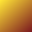#About Method Plugin

The Method plugin keeps a running tally of calculations expressed as a series of lines.

2 3 SUM

See Bottles of Beer Methods for examples.

### Lines

The first few characters determine how a line of a method is interpreted.

A line starting with digits defines a value and contributes that value to the contribution.

A line starting with words retrieves that value from nearby datasets and contributes it to the computation.

A line starting with all-caps calls out a computation to be applied to previous contributions.

Defined values and computation results are available to subsequent methods by the name that follows on the remainder of the line.

### Units

When the name of a defined value includes parenthesized words, these words are interpreted as units.

12 converts to (inches) from (feet) 3 converts to (feet) from (yards)

Some unit conversions can be applied automatically.

90 (inches) 9 (feet) SUM (yards) total

See Method Unit Conversions for more examples.

### Computations

All computations are defined in the apply function inside the method plugin. This limits the things you can say to compute.

Say SUM to sum the previous contributions.

Say PRODUCT to multiply the previous contributions.

Say AVERAGE to average the previous contributions.

Say MINIUM or MAXIUM to find extremes.

Say RATIO to find the ratio of two numbers.

Say ACCUMULATE to add to a running sum.

Say AVG, MIN or MAX as an acceptable abbreviation.

See Method Expressions for concise formulas.

650 (miles) 9.5 (hours) RATIO SHOW Average Speed

Say SHOW to display specific results in large digits while hiding other calculatons. (SHOW is otherwise like SUM)

### Application

The Method plugin exposes its computations such that they can be applied to new values by other plugins.

See About Rollup Plugin to see how calculations contributing to a dataset can be broken down into Methods on pages and then rolled back up to make a revised dataset.

See About Reduce Plugin to see how calculation on a specified sequence of pages can be treated as a whole and evaluated repeatedly as such.

### Programming

Additional computations may be added as needed by programmers for use on a specific site.

The plugin's apply function recognizes computations by name and performs the appropriate computation.

apply = (name, list, label) -> switch name when 'SUM' then sum list when 'AVG', 'AVERAGE' then avg list when 'MIN', 'MINIMUM' then _.min list when 'MAX', 'MAXIMUM' then _.max list when 'FIRST' then list when 'PRODUCT' then _.reduce list, (p,n)->p*=n when 'LOOKUP' then lookup list when 'POLYNOMIAL' then polynomial list, label else throw new Error "don't know how to #{name}"